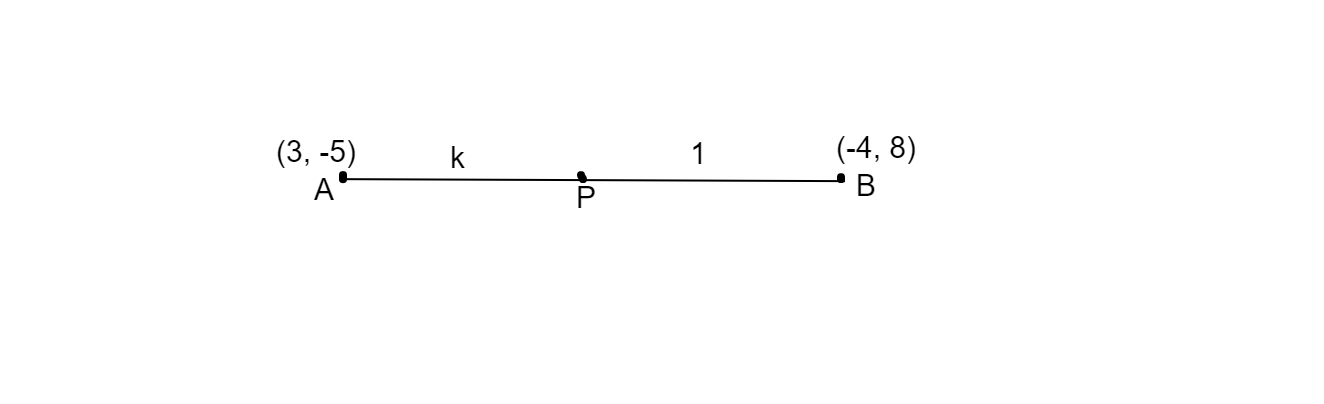# A point P divides the line segment joining the points$A( 3,\ -5)$ and $B( -4,\ 8)$ such that$\frac{AP}{PB} =\frac{K}{1}$ . if P lies on the line $x+y=0$, then find the value of K.

Given: A line segment joining two points $A( 3,\ -5)$  and $B( -4,\ 8)$, a point P on the line segment such that $\frac{AP}{PB} =\frac{K}{1}$ and P also lies on the line $x+y=0$.

To do: To find the value of k.

Solution: As known if there is a line segment joining two points two points $(x_{1} ,\ y_{1})$ and $( x_{2} ,\ y_{2} )$ and if the there is a point $P( x,\ y)$ dividing the line in the ratio m:n, then

$P( x,\ y) =\left(\frac{nx_{1} +mx_{2}}{m+n} ,\ \frac{ny_{1} +my_{2}}{m+n}\right)$here $m=k,\ n=1,\ x_{1} =3,\ y_{1} =-5,\ x_{2} =-4\ and\ y_{2} =8$

Using the division formula, we have

$P( x,\ y) =\left(\frac{-4k+3}{k+1} ,\ \frac{8k-5}{k+1}\right)$

$\Rightarrow x=\frac{-4k+3}{k+1} \ ,\ y=\frac{8k-5}{k+1}$         ...............$( i)$

$\because$ the point P lies on the line $x+y=0$,

on subtituting values of $( i)$ in the line $x+y=0$,

$\Rightarrow \frac{-4k+3}{k+1} +\frac{8k-5}{k+1} =0$

$\Rightarrow \frac{-4k+3+8k-5}{k+1} =0$

$\Rightarrow 4k-2=0$

$\Rightarrow 4k=2$

$\Rightarrow k=\frac{2}{4}$

$\Rightarrow k= \frac{1}{2}$